### Concept map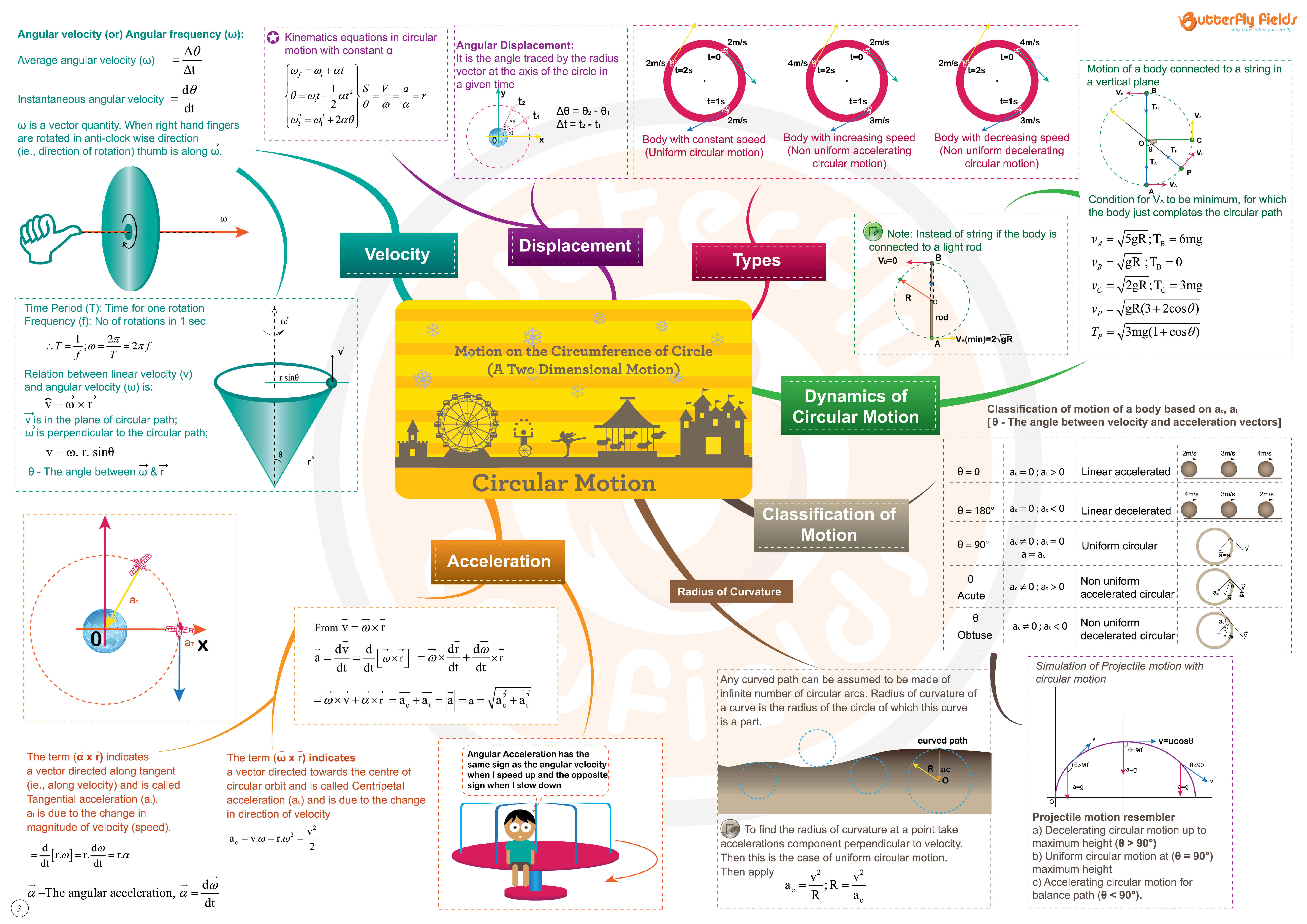A Concept map is a colorful, visual form of representing key concepts covered in a chapter . It can be used as an effective overview , learning and revision tool.

At its heart is a central topic of the chapter . This is then explored by means of branches representing main ideas, which all connect to this central idea.

Concept maps are metacognitive tools that give students awareness towards the process of learning and allow the representation of knowledge structures in thematic fields

### Questions

1) A rigid body is spinning with an angular velocity of 4 rad/sec, about an axis parallel to (3j-k) passing through the point (O) (i+3j-k). Find the velocity of the particle at the point ‘P’ (4i-2j+k) ?

2) Find the angular speed A relative to B, in both the cases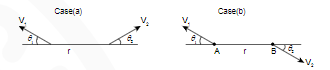3) A bob attached to a light string is oscillating in a vertical plane. It’s acceleration at extreme and mean position will be equal, for what value of the angular amplitude (θ)?

4) A point moves with deceleration along the circle of radius R so that at any time, its at, ac are equal in magnitude. If at t = 0, velocity of the point = V0, then express its velocity as a function of time (t) and distance covered (S)?

5) A particle moves uniformly with speed ‘u’ along a parabolic path y = bx2, where ‘b’ is a positive constant. Find the acceleration of the particle and radius of curvature of the path at x =0 ?

6) The velocity and acceleration of a particle moving on a circular path are given by, V = (3i + 2j) m/sec, a = (-4i + 2j) m/sec2. What nature of motion it is ?

7) A particle starts moving on a circle of radius ‘R’ and has a centripe- -tal acceleration ‘a’. It’s centripetal and tangential acceleration will be equal after what time ?

8) A body starts moving on the circumference of the circle of radius √2 mt, with constant angular acceleration of π/4 rad/sec2. Find the average velocity as it completes quarter circle?

9) A bob is attached to one end of a string, other end of which is fixed at Peg ‘A’. The bob is taken to the position shown in figure, from there it is projected with minimum speeds Vc, Va in clockwise and anti clockwise directions so as hit a Peg B. What is the ratio betw- -een Vc and Va ?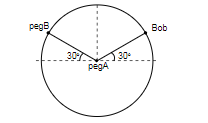### A comprehensive revision tool for competitive exams

JEE (Mains), BITSAT, VITEE, KCET, MHCET, EAMCET, TANCET, MP PET, GUJCET, AIPMT, JIPMER, AFMC & Board Exams

“Easy way to interlink all key concepts without getting distracted by unnecessary details. Helps in quick review and fast revision.” - Arvind Saraf, JEE AIR 001

• Revise like never before!
• One topic in just one concept map
• Colored themes for grasp better understanding

### Shop Our Concept Map Books Collection### TinkL Electronics & Robotics Kit | Make 40+ Home Automation Projects 100+ parts with Sensors | No Coding Required

Rs. 4,799.00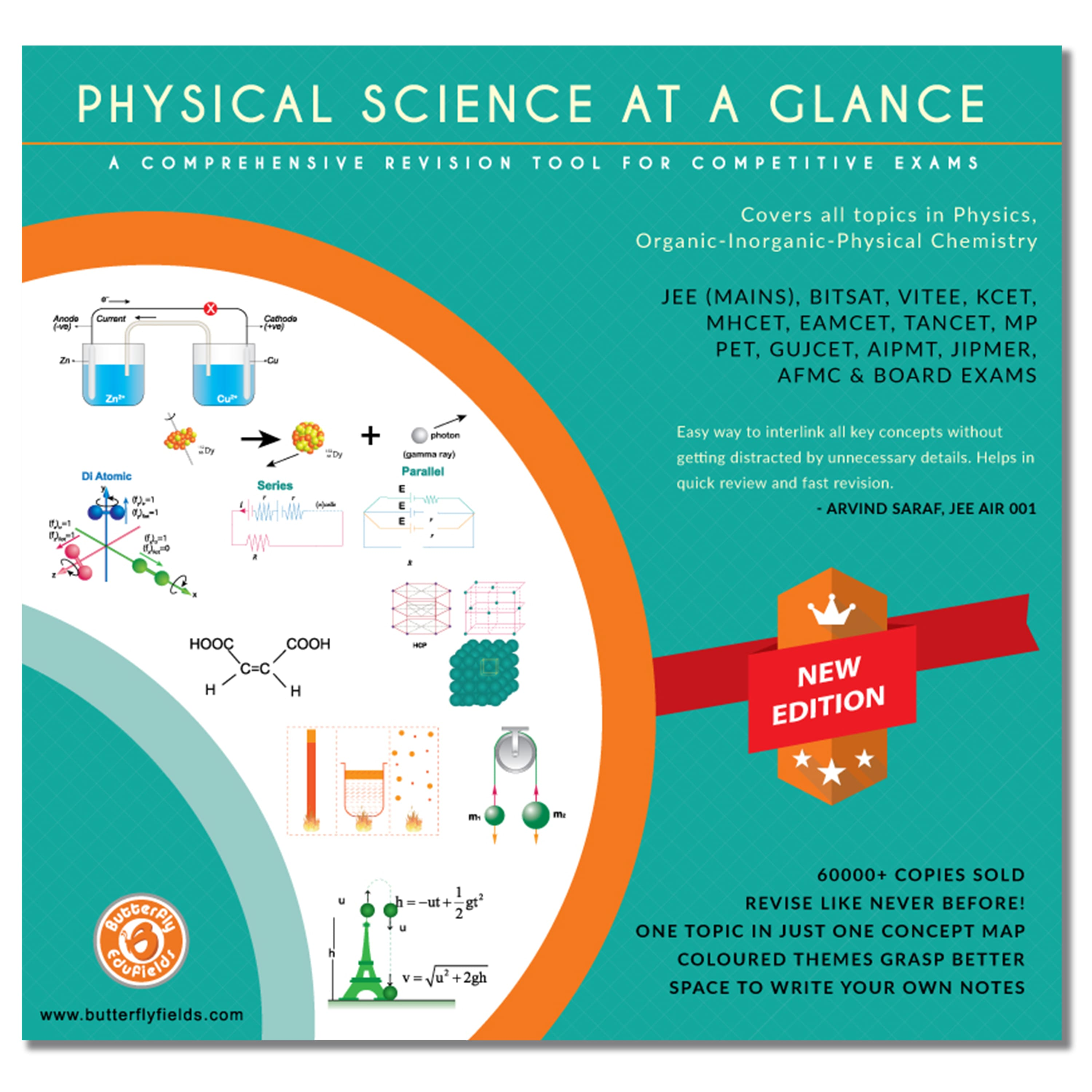### Physics & Chemistry Combined Concept Map Book | Effective revision for NEET IIT JEE Mains, Advanced & NCERT class 11, 12

Rs. 749.00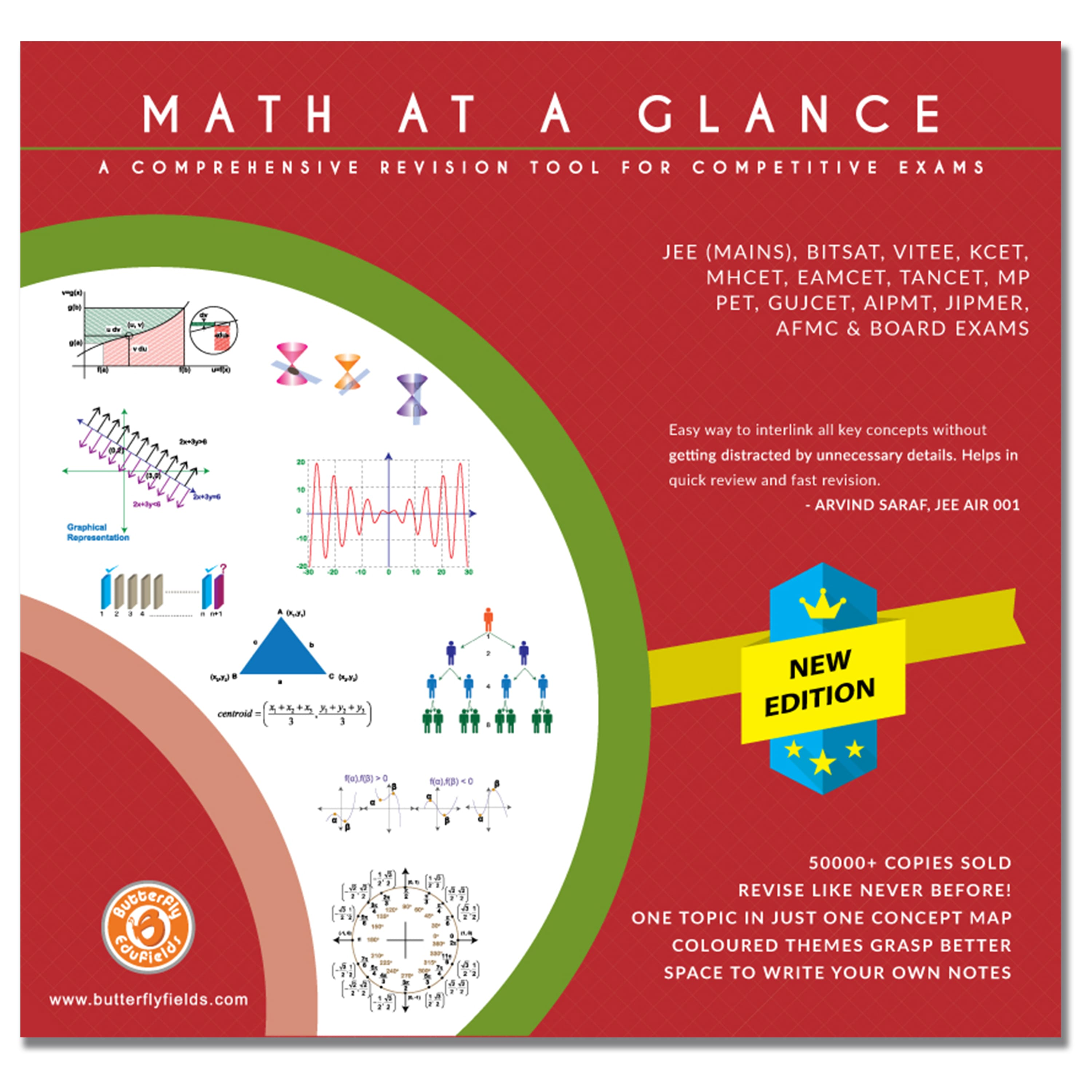### Mathematics Combined Concept Map Book | Effective revision for NEET IIT JEE Mains, Advanced & NCERT class 11, 12

Rs. 399.00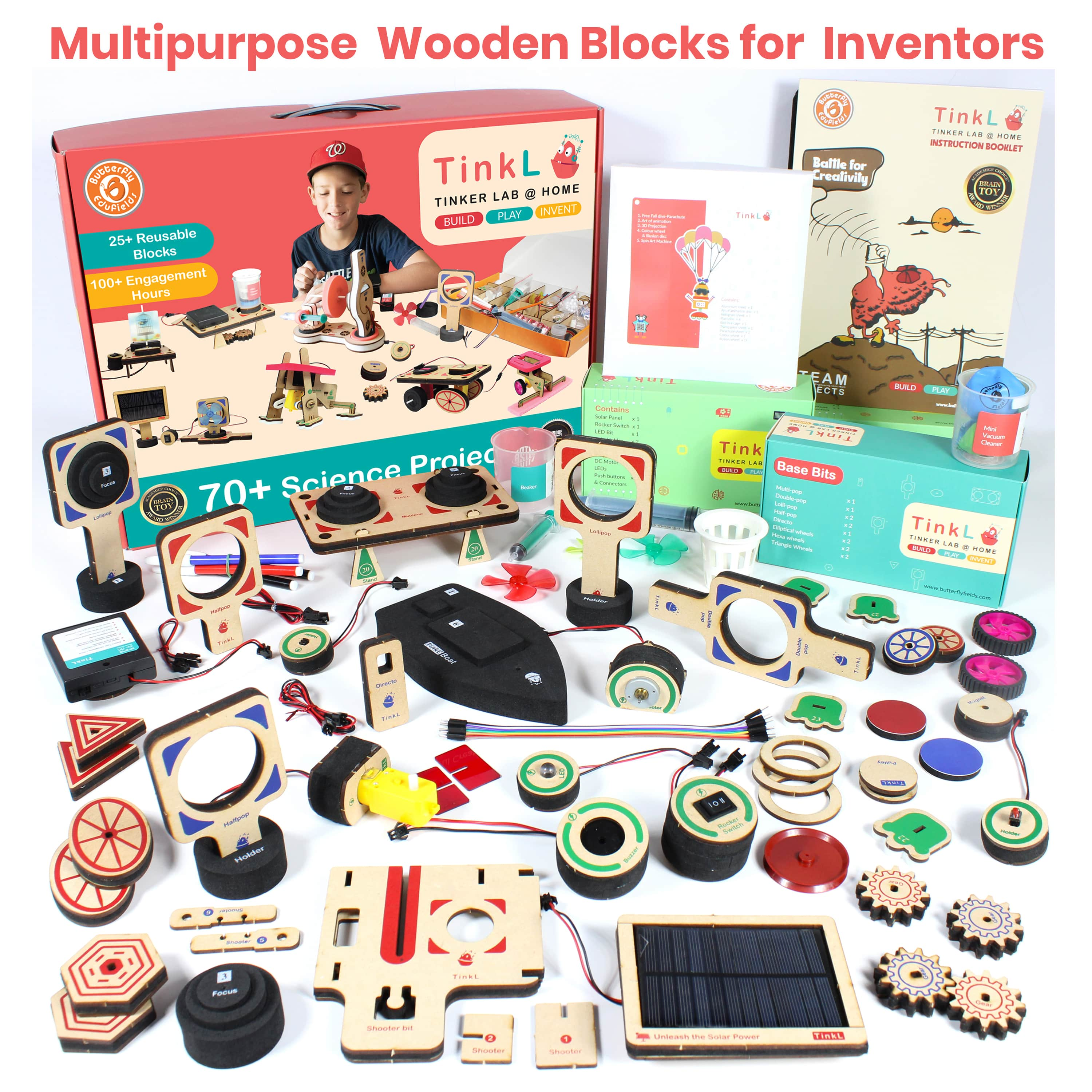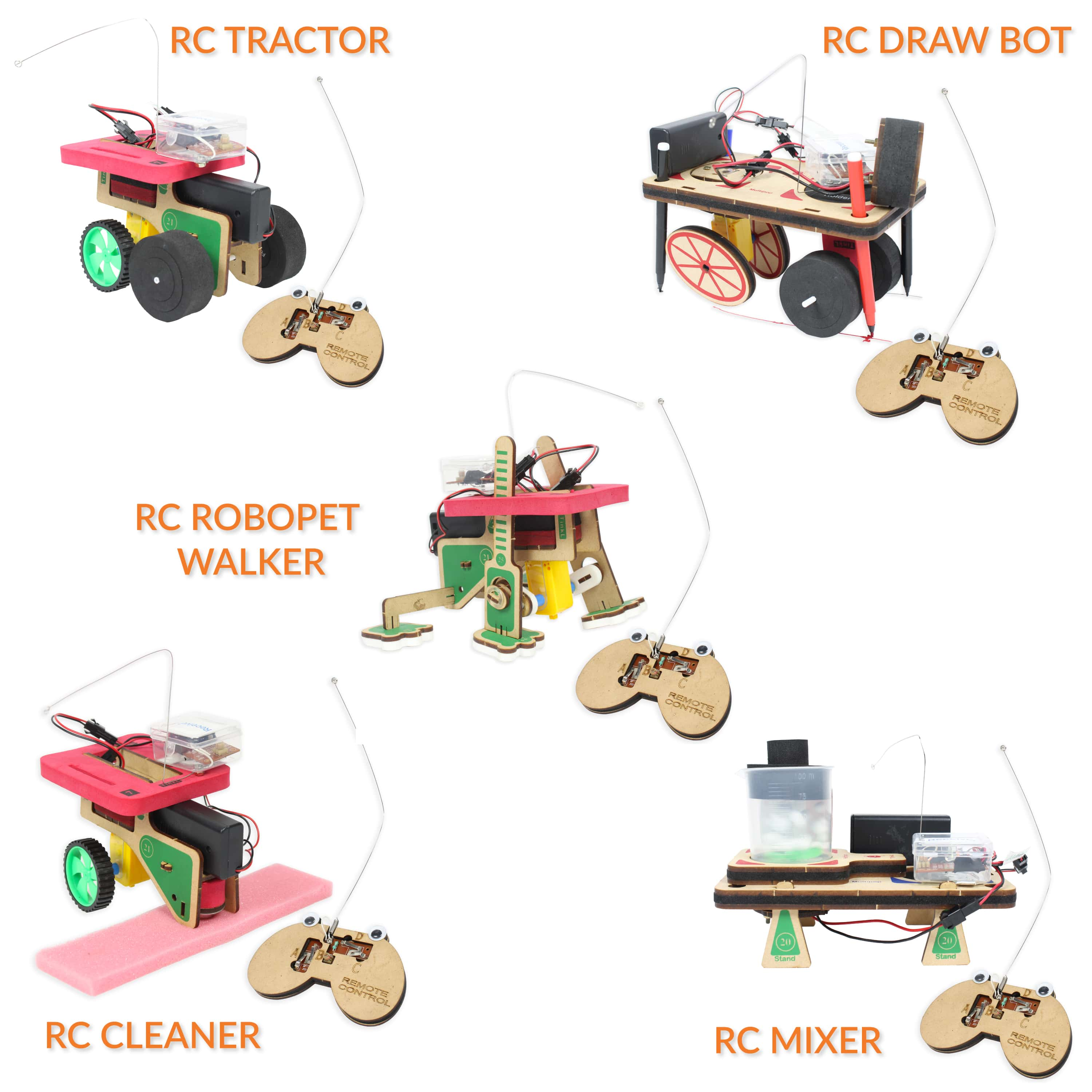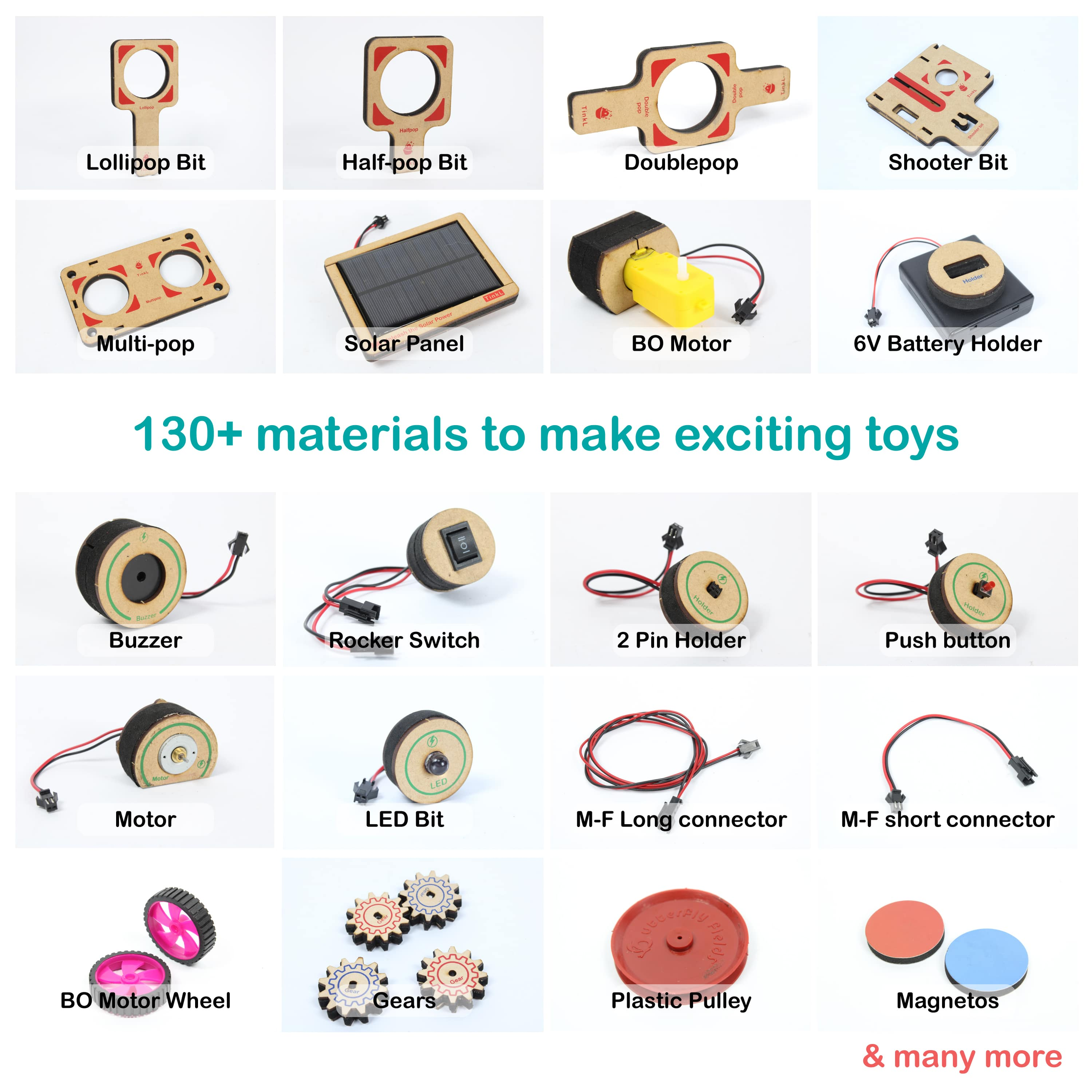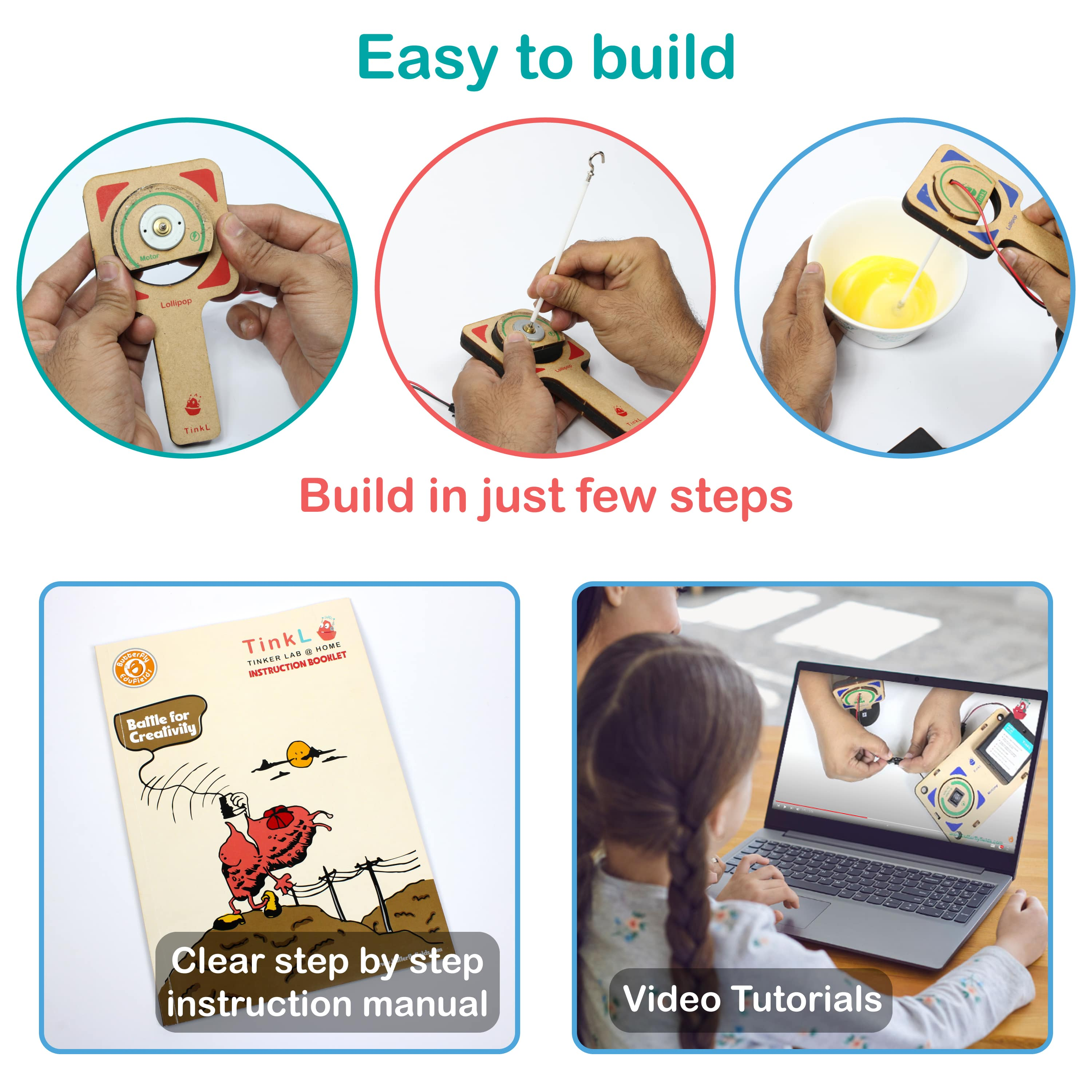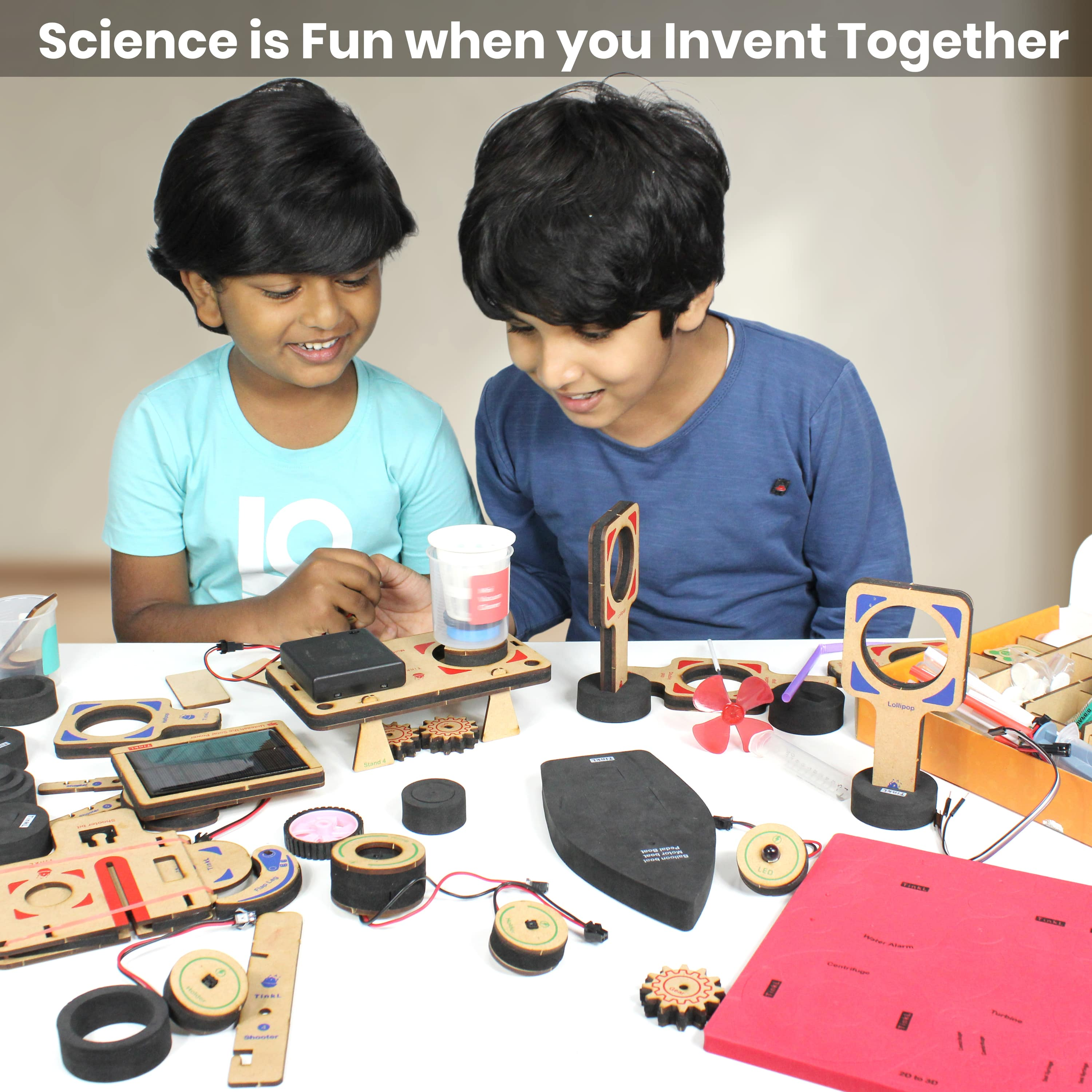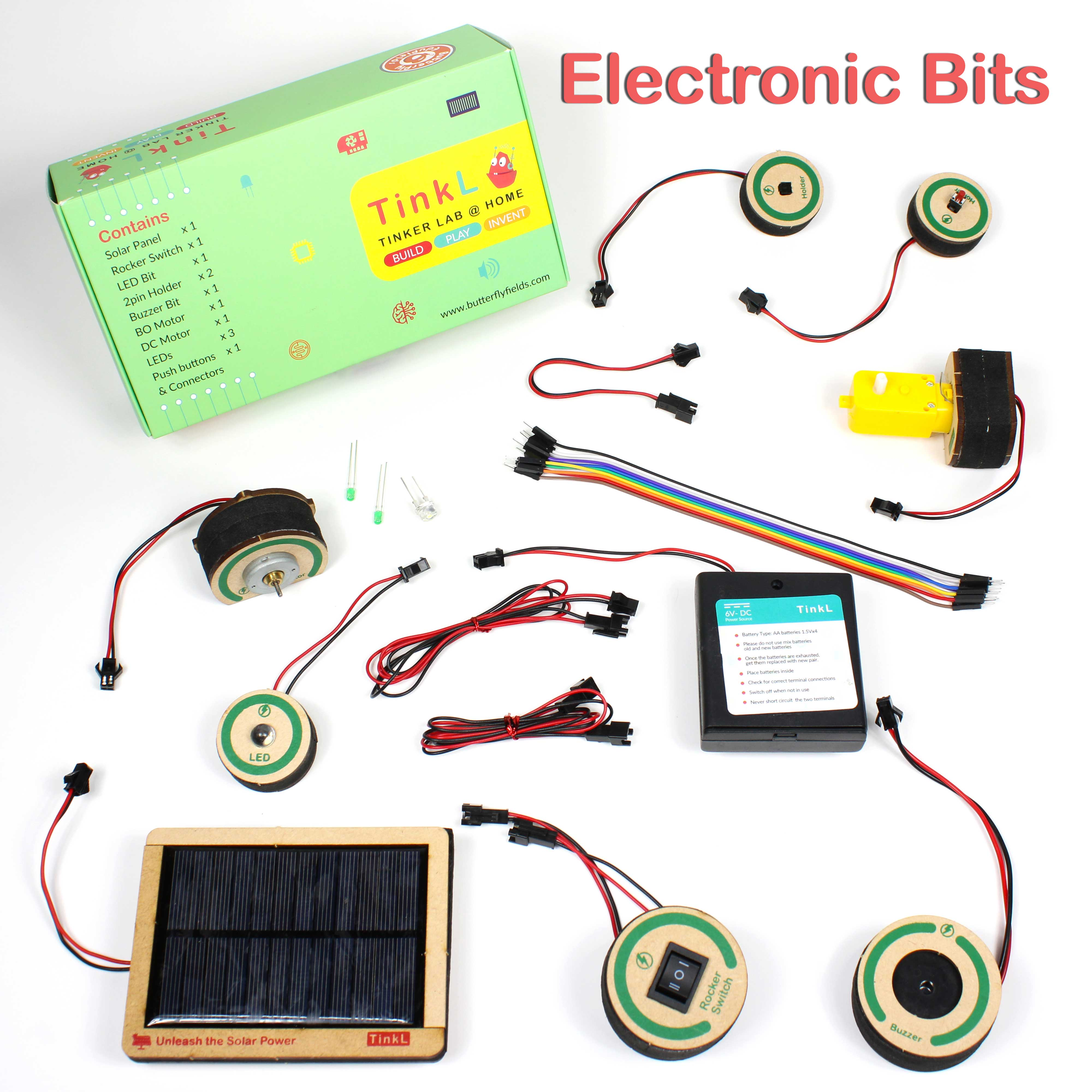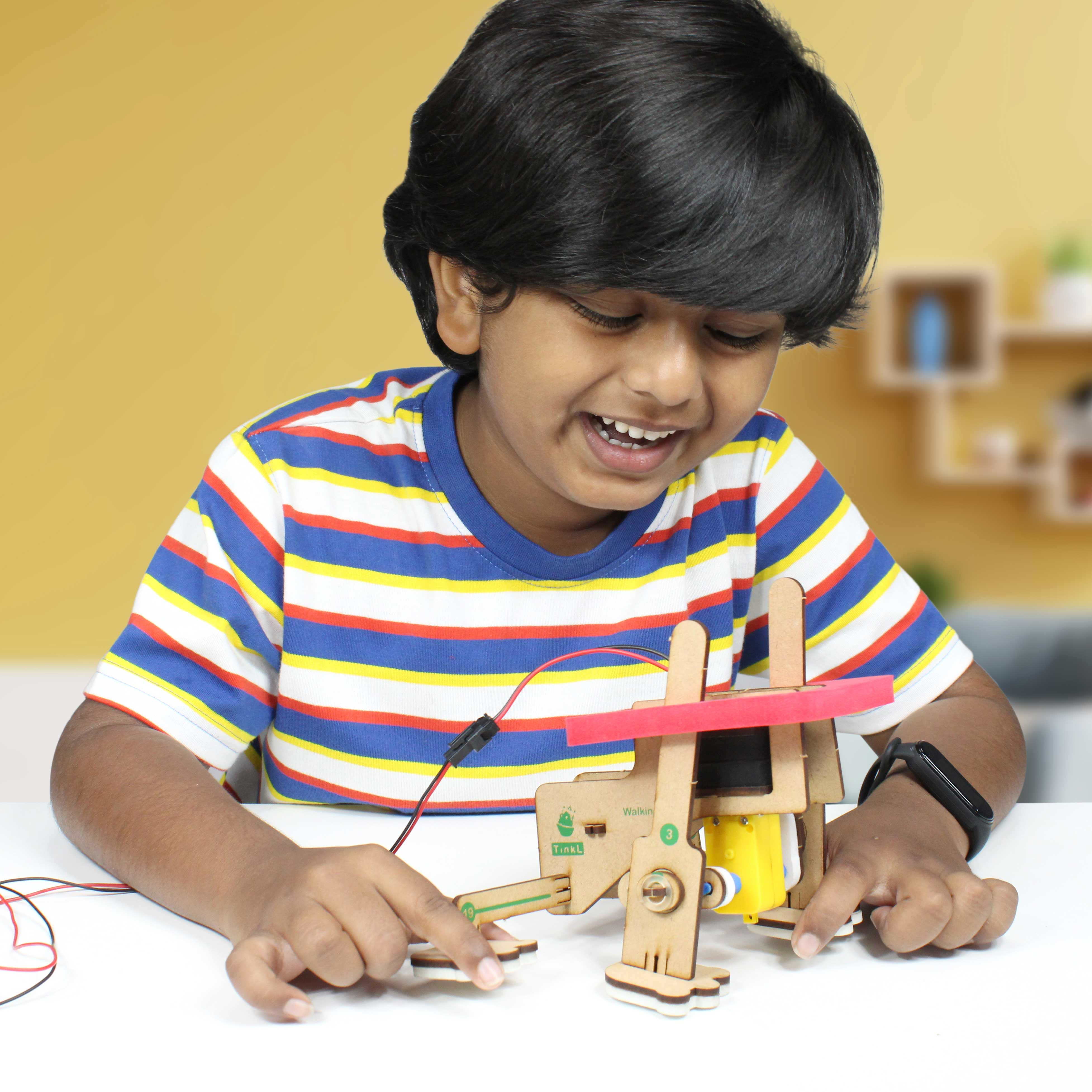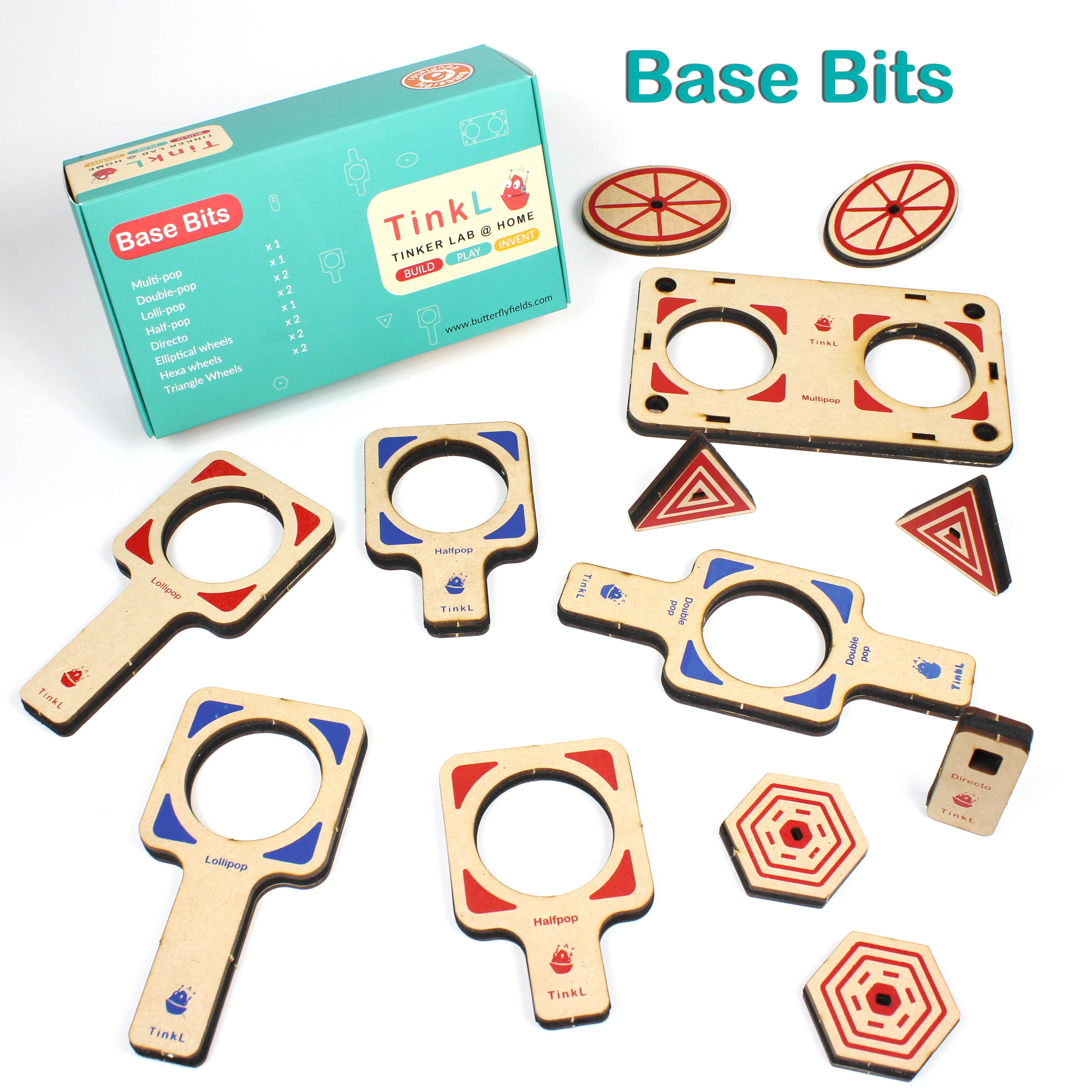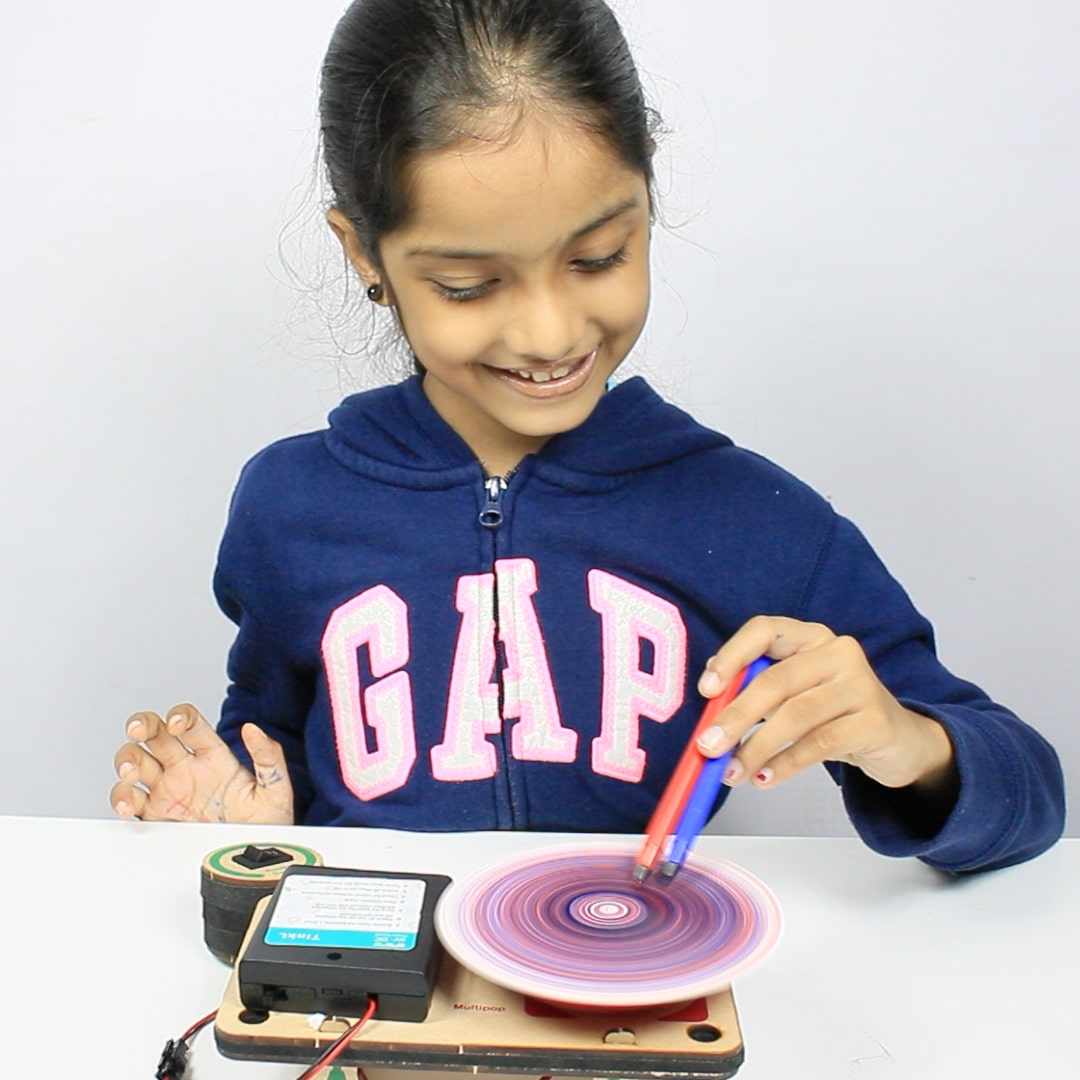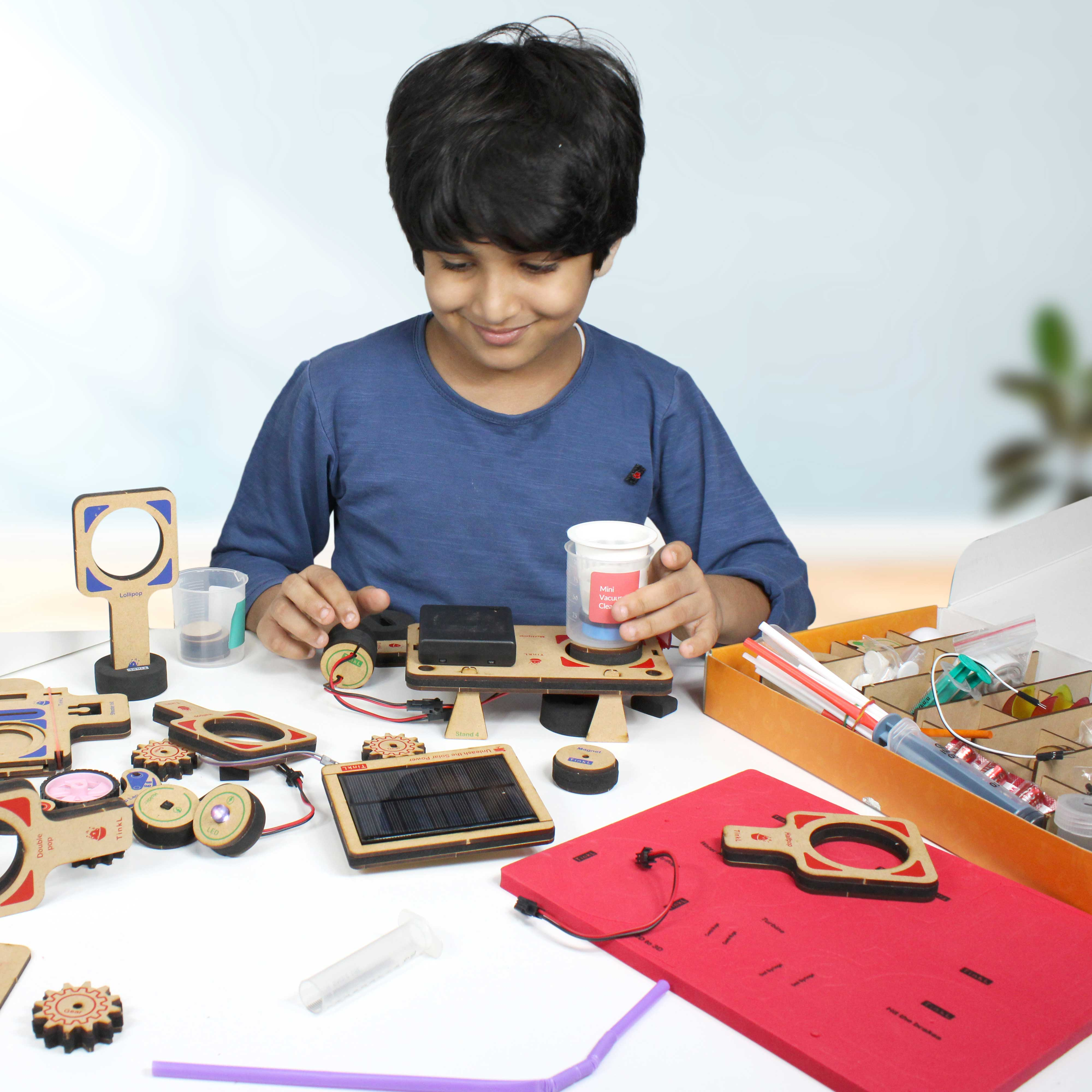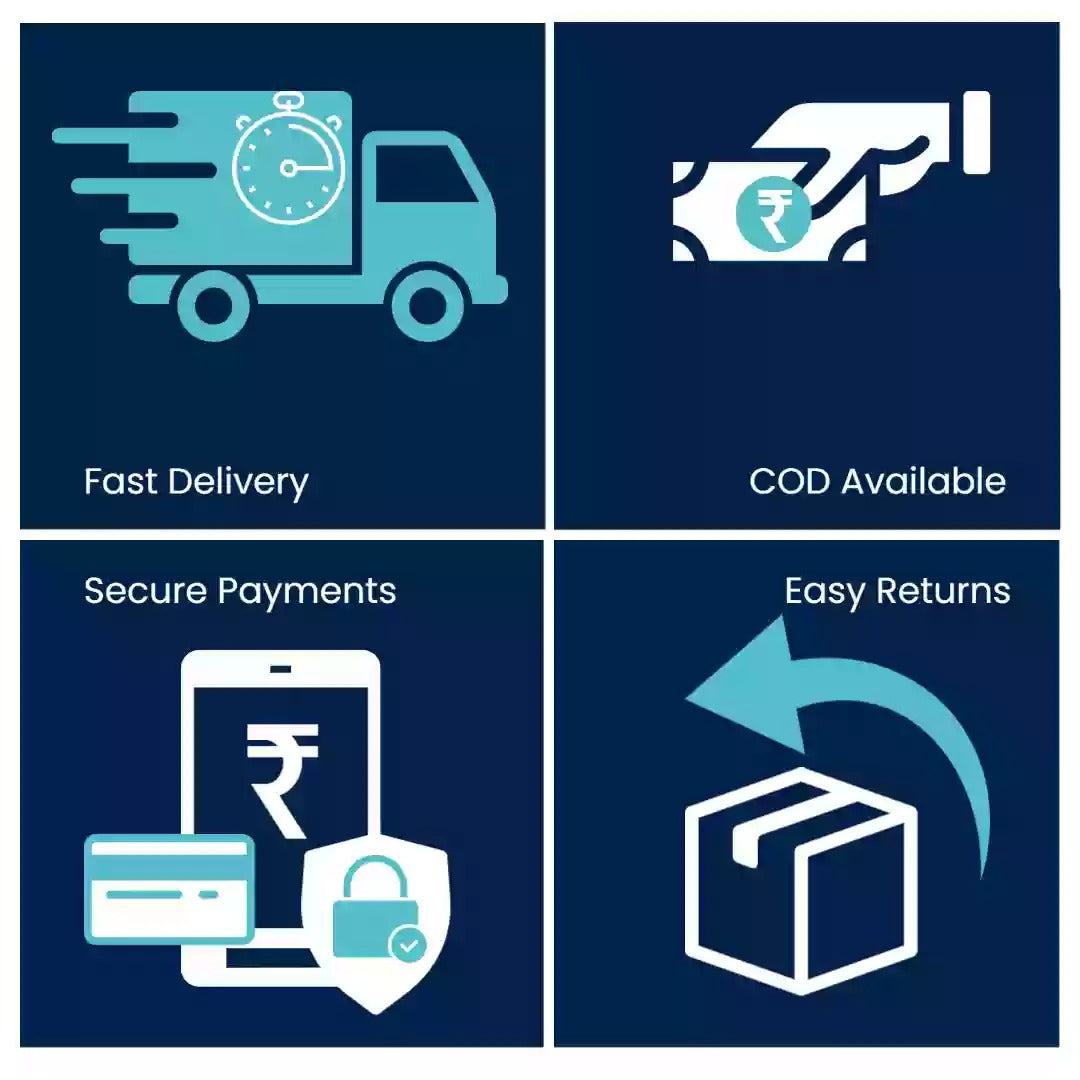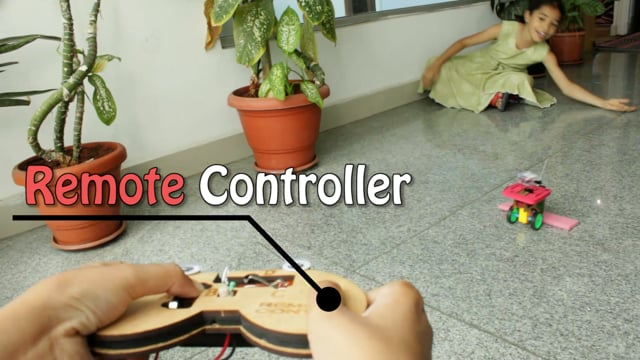Rs. 4,999.00

### Explore all topics

• Kinematics
• Projectile Motion
• Circular Motion
• Vectors
• Force
• Work Power Energy
• Centre Of Mass
• Law of Conservation of Momentum
• Gravitation
• Rotational Motion
• Simple Harmonic Motion
• Properties of matter and fluid mechanics
• Thermometry
• First Law of Thermo dynamics
• Second law of thermo dynamics
• Sound waves
• Ray optics
• Wave optics
• Electro Statics
• Current Electricity
• Thermal and Magnetic effects of Electric current
• EM Induction & AC circuits
• Nuclear Physics
• EM Waves & Communication Devices
• Semi conductor devices
Sunday,Monday,Tuesday,Wednesday,Thursday,Friday,Saturday
January,February,March,April,May,June,July,August,September,October,November,December
Not enough items available. Only [max] left.